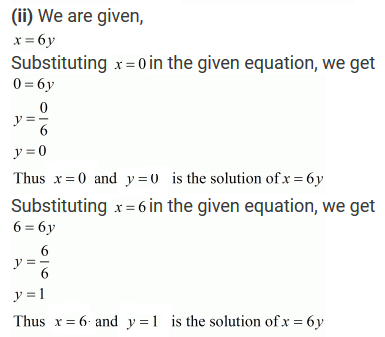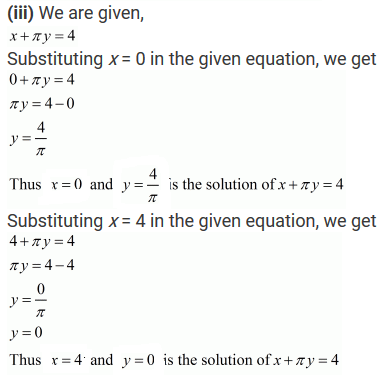#### Chapter 13 Linear Equations in Two Variables R.D. Sharma Solutions for Class 9th Exercise 13.2

Exercise 13.2

1. Write two solutions for each of the following equations:
(i) 5x–2y = 7
(ii) x = 6y
(iii) x + πy = 4
(iv) 2/3x – y = 4.

Solution2. Write two solutions of the form x = 0, y = a and x = b, y = 0 for each of the following equations:

(i) 5x − 2y = 10
(ii) -4x + 3y = 12
(iii) 2x + 3y =24

Solution

(i) We are given,

5x – 2y = 10
Substituting x = 0 in the given equation,
We get;
5×0 – 2y = 10
– 2y = 10
– y = 10/2
y = – 5
Thus x =0 and y = -5 is the solution of 5x-2y = 10
Substituting y = 0 in the given equation, we get 5x —2 x 0 = 10
5x = 10
x = 10/2
x = 2
Thus x =2 and y = 0 is a solution of 5x-2y = 10

(ii) We are given, – 4x + 3y = 12

Substituting x = 0 in the given equation,
we get;
-4 × 0 + 3y = 12
3y = 12
y = 4
Thus x = 0 and y = 4 is a solution of the -4x + 3y = 12
Substituting y = 0 in the given equation, we get;
- 4 x + 3 × 0 = 12
- 4x = 12
x = -12/4
x = -3
Thus x = -3 and y =0 is a solution of -4x + 3y = 12

(iii) We are given, 2x + 3y = 24

Substituting x = 0 in the given equation, we get;
× 0 + 3y = 24
3y =24
y = 24/3
y = 8
Thus x = 0 and y = 8 is a solution of 2x+ 3y = 24
Substituting y = 0 in the given equation, we get;
2x +3 × 0 = 24
2x = 24
x = 24/2
x = 12
Thus x =12 and y = 0 is a solution of 2x + 3y = 24

3. Check which of the following are solutions of the equations 2x - y = 6 and which are not:

Solution

We are given, 2x – y = 6

(i) In the equation 2 x – y = 6,
We have L.H.S = 2x - y and R.H.S = 6
Substituting x = 3 and y = 0 in 2x - y ,
We get L.H.S = 2 x 3 – 0 = 6
⇒ L.H.S = R.H.S
⇒ (3,0) is a solution of 2x - y = 6.

(ii) In the equation 2x - y = 6,

We have L.H.S = 2x- y and R.H.S = 6
Substituting x = 0 and y = 6 in 2x - y
We get L.H.S = 2 × 0 - 6 = -6
L.H.S R.H.S
⇒ (0,6) is not a solution of 2x - y = 6.

(iii) In the equation 2x - y = 6,

We have L.H.S = 2x - y and R.H.S = 6
Substituting x = 2 and y = - 2 in 2x - y,
We get L.H.S = 2 × 2 - (-2) = 6
⇒ L.H.S = R.H.S
⇒ (2,-2) is a solution of 2x - y = 6.

(iv) In the equation 2x-y = 6, we have
LHS = 2x-y and RHS = 6
Substituting x = √3 and y = 0 in 2x - y, we get
LHS = 2×√3-0 = 2√3
LHS ≠ RHS
(√3, 0) is not the solution of 2x - y = 6.

(v) In the equation 2x - y = 6, we have
LHS = 2x-y and RHS = 6
Substituting x = 1/2 and y = -5 in 2x-y, we get
LHS = 2× 1/2 and y = -5 in 2x-y, we get
LHS = RHS
(1/2, -5) is the solution of 2x-y = 6.

4. If x = −1, y = 2 is a solution of the equation 3x + 4y = k, find the value of k.

Solution

We are given, 3 x + 4 y = k

Given that, (-1,2) is the solution of equation 3x + 4y = k.
Substituting x = -1 and y = 2 in 3x + 4y = k,
We get; 3x – 1 + 4 × 2 = k
k = -3 + 8
k = 5

5. Find the value of λ, if x =-λ and y=5/2 is a solution of the equation x+4y-7=0.

Solution6. If x = 2α + 1 and y = α − 1 is a solution of the equation 2x − 3y + 5 = 0, find the value of α.

Solution

We are given, 2x -3y +5 = 0

(2a + 1, a – 1 ) is the solution of equation 2x – 3y + 5 = 0.
Substituting x = 2a + 1 and y = a – 1 in 2x – 3y + 5 = 0,
We get 2 x 2a + (1- 3) x a – 1 + 5 = 0
⇒ 4a + 2 – 3a + 3 + 5 = 0
⇒ a + 10 = 0
⇒ a = – 10

7If x=1 and y=6 is a solution of the equation 8x-ay+a2=0. Find the value of a.

Solution

Given,
8x - ay + a2 = 0
(1, 6) is the solution of equation 8x-ay+a2 = 0
Substituting, x = 1 and y = 6 in 8x-ay+a2 = 0, we get
8×1 - a×6 +a2 = 0
⇒ a2 - 6a + 8 = 0